## Before you start…

Before starting this worksheet, you should have completed all three previous worksheets of the Very Brief Guide to R. Once you have, you’ll have an R project that contains a script like this:

## Exploring data
library(tidyverse)
cpsdata %>% summarise(mean(income))
cpsdata %>% summarise(mean(hours, na.rm = TRUE))

## Grouping data: worksheet
cpsdata %>% group_by(sex) %>% summarise(mean(income))
cpsdata %>% ggplot(aes(income, colour=factor(sex))) + geom_density(aes(y=..scaled..))
cpslow <- cpsdata %>% filter(income < 150000)

## Grouping data: exercise
cpslow %>% ggplot(aes(income, colour=factor(sex))) + geom_density(aes(y=..scaled..))
cpslow %>% group_by(native) %>% summarise(mean(income))
cpslow %>% ggplot(aes(income, colour=factor(native))) + geom_density(aes(y=..scaled..))

## Evidence Part 1
library(BayesFactor, quietly = TRUE)
ttestBF(formula = income ~ sex, data = data.frame(cpsdata))
t.test(cpsdata$income ~ cpsdata$sex)

## Evidence Part 1: Exercise
ttestBF(formula = income ~ native, data = data.frame(cpslow))
t.test(cpslow$income ~ cpslow$native)

## Contents

We’re going to use some new data in this final worksheet, so download it from here and upload it to RStudio, and then load the data into a dataframe called gdata. Look at the Exploring data worksheet if you need a reminder on how to do this. If you’ve done it correctly, you’ll get an output like this:


── Column specification ────────────────────────────────────────────────────────
cols(
grp = col_double(),
ingroup = col_double(),
outgroup = col_double(),
dominance = col_double()
)

Next, click on gdata in the Environment window, and take a look. The data is from an experiment where each of 25 groups of people selected a leader and then completed a task together. Afterwards, they answered some questions about their group. Specifically, they rated their ingroup closeness (how close, psychologically speaking, they felt to members of their own group), their outgroup distance (how distant they felt from members of other groups), and how dominant their group leader was in their group. The ratings were made inidividually, and then averaged to give one number per group per measure.

Here’s what each of the column labels mean:

Column Description Values
grp ID number of the group a number
ingroup Group’s mean rating of ingroup closeness 1 (low) - 10 (high)
outgroup Group’s mean rating of outgroup distance 1 (low) - 10 (high)
dominance Group’s mean rating of the dominance of their group leader 1 (low) - 10 (high)

This is a small dataset comprising 25 groups.

## Scatterplots

One question we can ask about these data concerns the relationship between ingroup closeness and outgroup distance.

For example, does high ingroup closeness tend to be associated with high outgroup distance – perhaps feeling close to your ingroup is associated with feeling distant from your outgroup?

Or perhaps high ingroup closeness is associated with low outgroup distance — feeling close to your own group also makes you feel close to other groups? Or, a third option, perhaps the two things are unrelated — whether you have high or low ingroup closeness does not predict your outgroup distance.

One way to look at this question is to produce a scatterplot. On a scatterplot, each point represents one group. That point’s position on the x-axis represents their ingroup closeness, and that point’s position on the y-axis represents their outgroup distance.

The command to produce a scatterplot in R is much like the command for a density plot. It is:

gdata %>% ggplot(aes(x = ingroup, y = outgroup)) + geom_point()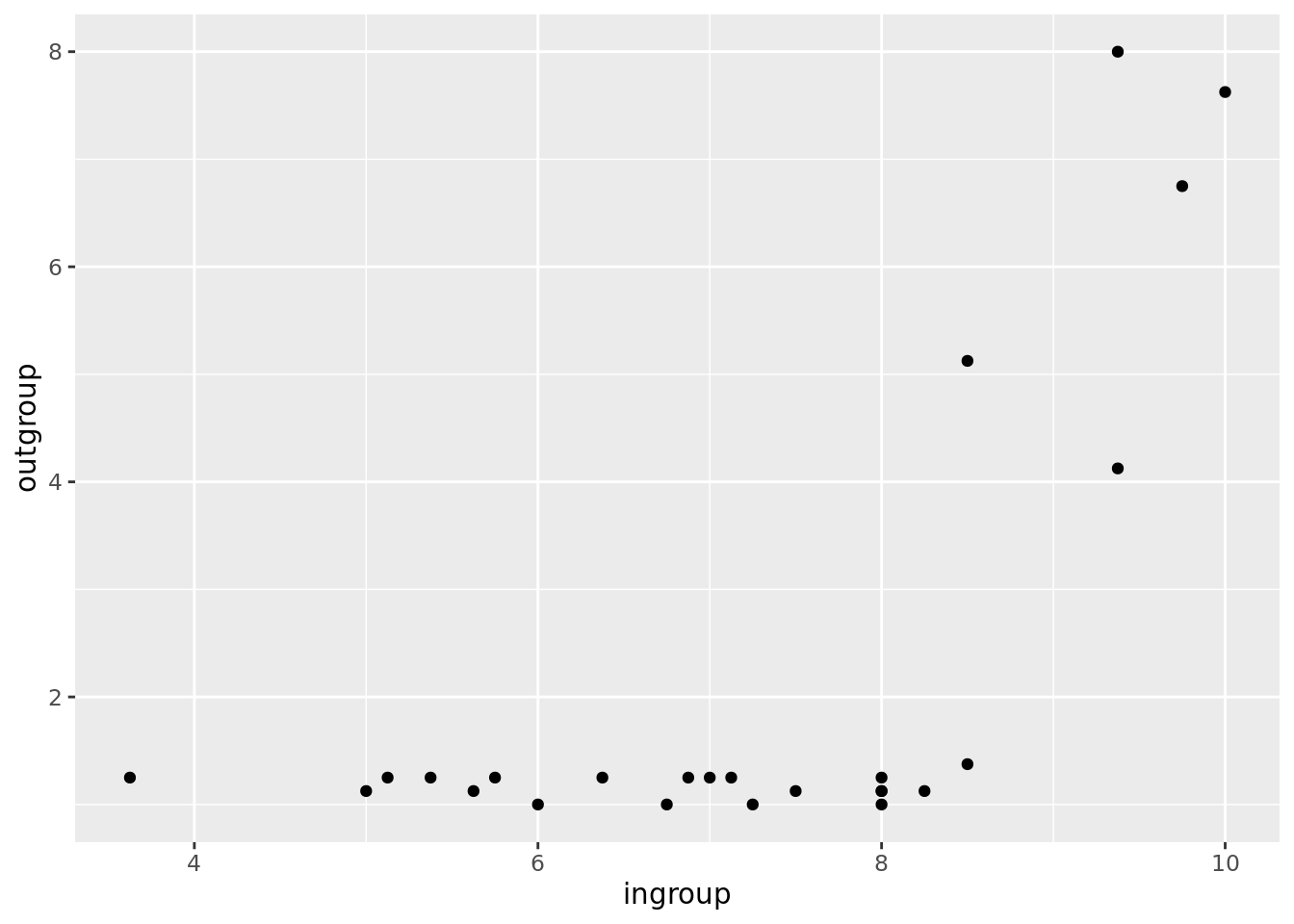### Explanation of command

The command takes the data from the gdata dataframe, and pipes it (%>%) to ggplot to produce a graph. The rest of the command tells ggplot what type of graph we want:

geom_point() - We want a scatterplot

aes(x = ingroup, y = outgroup) - We want the variable ingroup on the x-axis, and the variable outgroup on th y-axis.

### Discussion of output

In the above scatterplot, many of the points are close to the x axis. This is becasue, as we saw above, most groups gave a rating close to 1 for outgroup distance. However, once we get to an ingroup closeness above 8, an interesting pattern starts to emerge. As ingroup closeness increases from 8 to 10, outgroup distance rises from around 1 to around 7 or 8.

So it seems that, in this example dataset, ingroup closeness and outgroup distance are related. We call this type of relationship a correlation.

## Measuring correlation

Sometimes, it’s useful to have a single number that summarises how well two variables are correlated. We can calculate this number, called a correlation co-efficient, using the cor command in R:

cor(gdata$ingroup, gdata$outgroup)
 0.6641777

### Explanation of command

Here’s what each part of the command means:

cor() - The command to calculate a correlation co-efficient.

gdata$ingroup - One variable is in the ingroup column of the gdata data frame. , - this comma needs to be here so R knows where one variable ends and the other begins. gdata$outgroup - The other variable is in the outgroup column of the gdata data frame.

### Explanation of output

In the above example, the correlation co-efficient was about 0.66. By tradition, we use a lower case r to represent a correlation co-efficient, so here r = 0.66. In order to make sense of this number, you need to know that the biggest r can ever be is 1, and the smallest it can ever be is -1.

Where r = 1: A correlation of 1 means a perfect linear relationship. In other words, there is a straight line you can draw that goes exactly through the centre of each dot on your scatterplot. The line can be shallow, or steep. Here are some examples: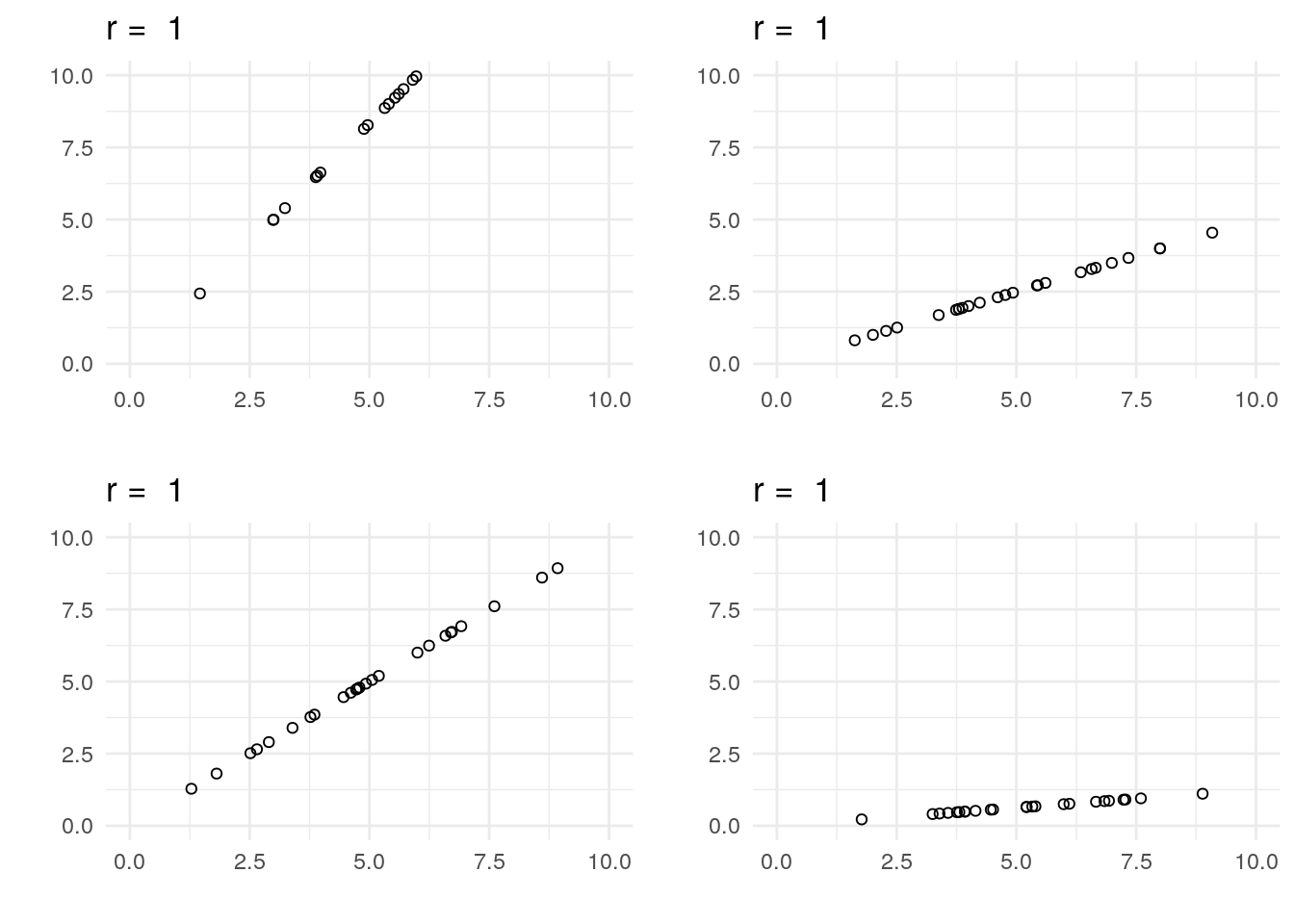Where r = 0: A correlation of zero means there is no relationship between the two variables. Here are some examples: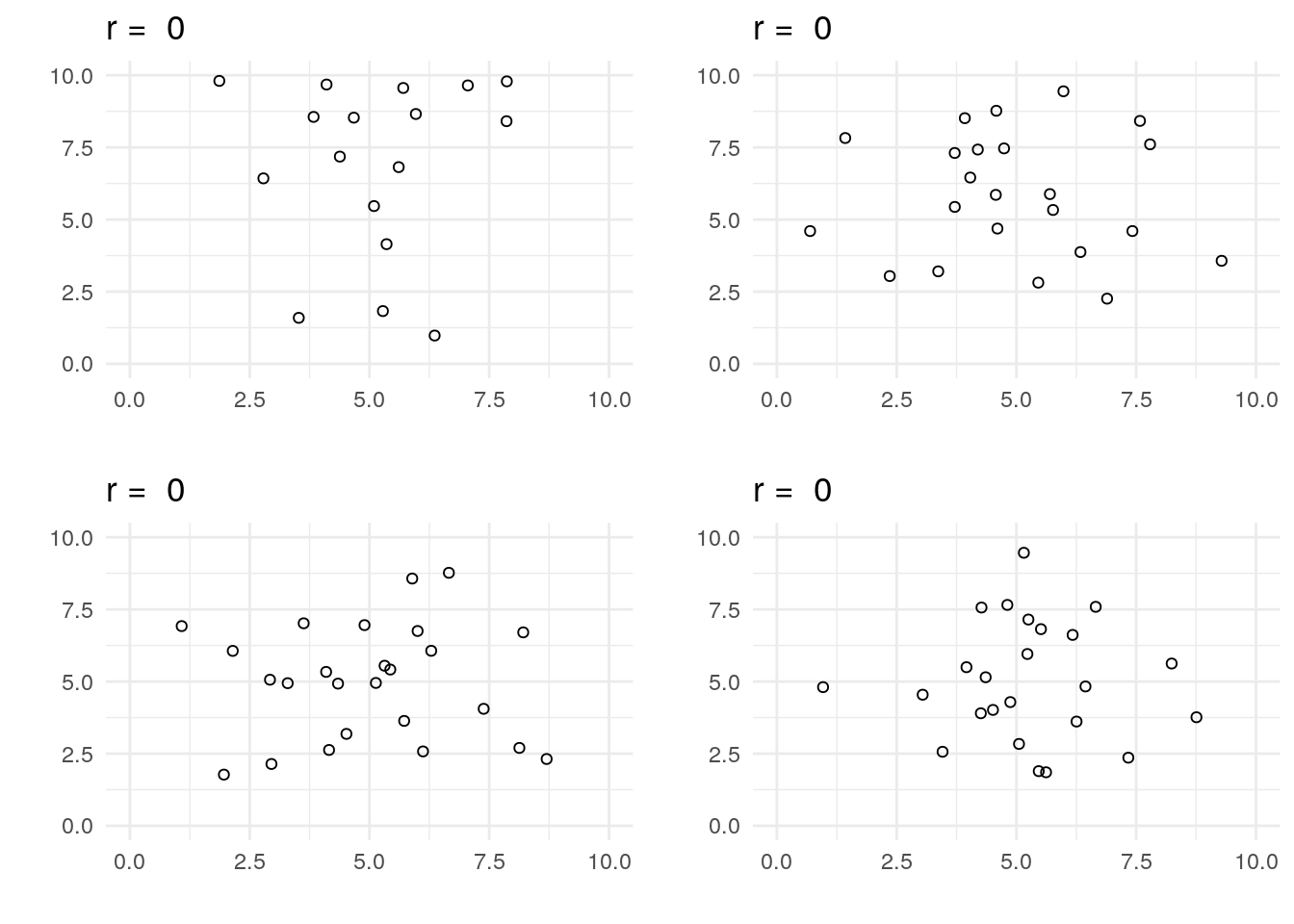Where r is between 0 and 1: As the correlation co-efficient gets further from zero, the relationship between the two variables becomes more like a straight line. Here are some more examples: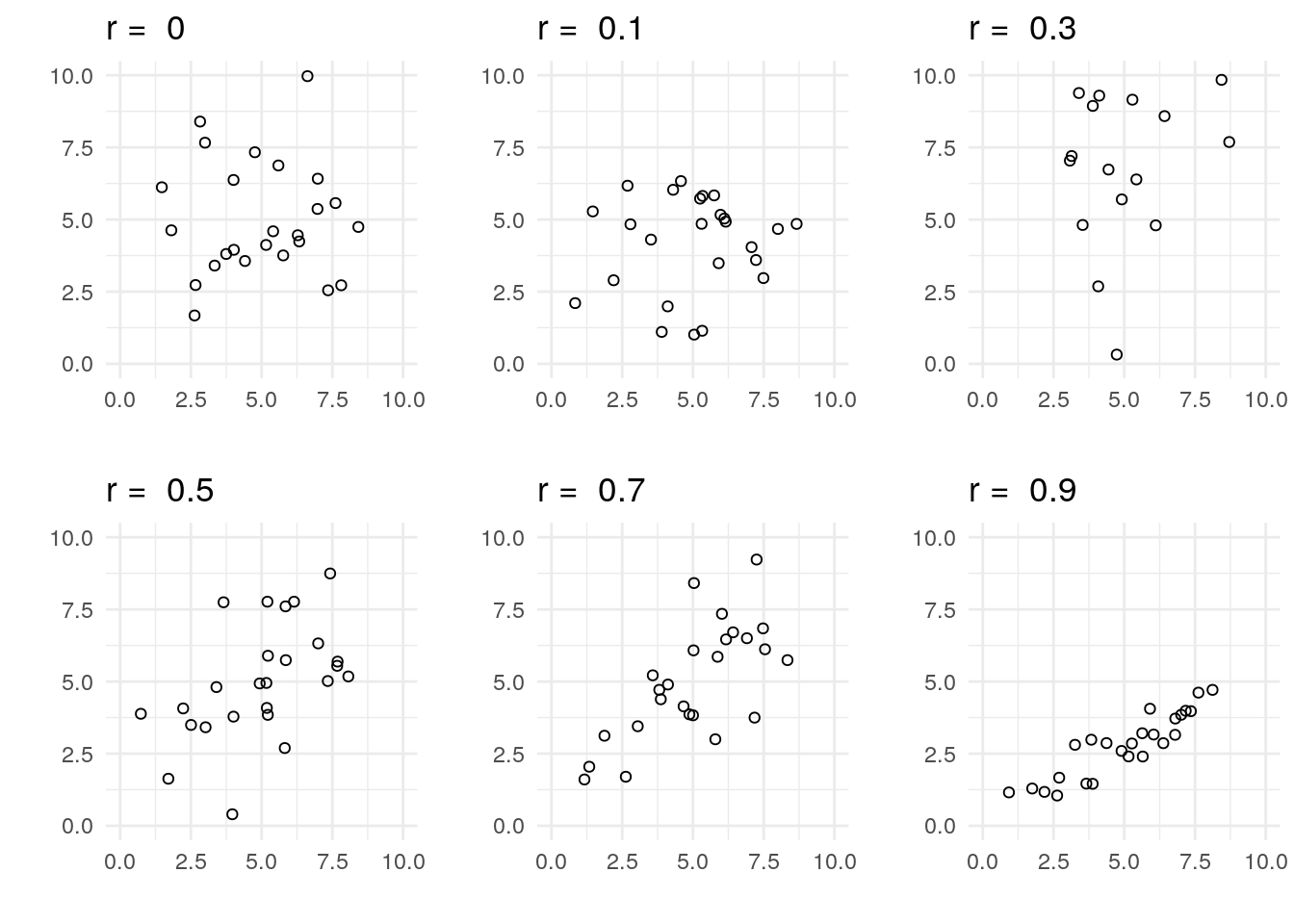Where r is less than 0: A negative correlation co-efficient just means that, as one variable gets larger, the other gets smaller: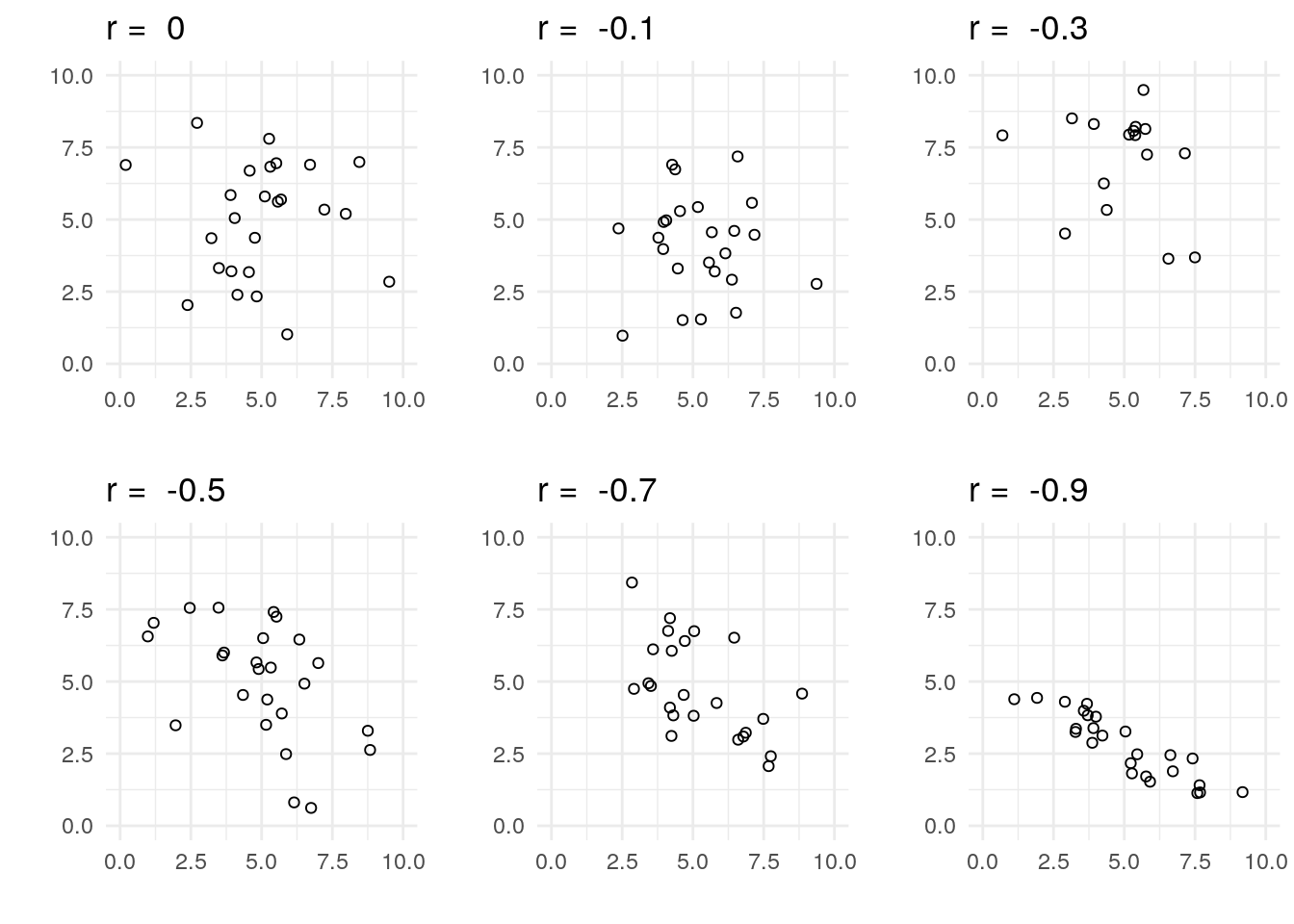## Evidence for correlation

So far, we’ve produced a scatterplot of ingroup closeness versus outgroup distance, and we’ve calculated a correlation co-efficient for that relationship ( r=0.66 in the example above). But is the relationship between these two variables real, or a fluke? Much like the Bayesian t-test we calculated in the previous worksheet, we can calculate a Bayes Factor for the relationship between two variables. This uses the same BayesFactor package, which we already loaded in the last worksheet.

To calculate a Bayes Factor for the correlation, we use the correlationBF command, which has a similar format to the cor command above:

correlationBF(gdata$ingroup, gdata$outgroup)
Bayes factor analysis
--------------
 Alt., r=0.333 : 89.70525 ±0%

Against denominator:
Null, rho = 0
---
Bayes factor type: BFcorrelation, Jeffreys-beta*

The Bayes Factor is reported on the third line, towards the right. In this example, our Bayes Factor is about 89.7. This means it’s about ninety times as likely there is a relationship between these two variables as there isn’t. This is larger than the conventional threshold of 3, so psychologists will generally believe you when you claim that there is a relationship between ingroup closeness and outgroup distance. If the Bayes Factor had been less than 0.33, this would have been evidence that there was no relationship.

As we covered in the Evidence worksheet, historically psychologists have typically reported p values, despite the fact that p values are widely misinterpreted. If you want to calculate a p value for a correlation co-efficient, you can use the following command:

cor.test(gdata$ingroup, gdata$outgroup)

## Exercise

In this exercise, you’ll apply what you’ve learned to the relationship between ingroup closeness, and group-leader dominance. Do each of the following analyses:

1. Make a scatterplot with ingroup closeness on the x-axis, and group-leader dominance on the y-axis.

2. Calculate the correlation co-efficient for ingroup versus dominance.

3. Calculate the Bayes Factor for this correlation.

### Expected output

If you’ve done it right, these are the answers you’ll get: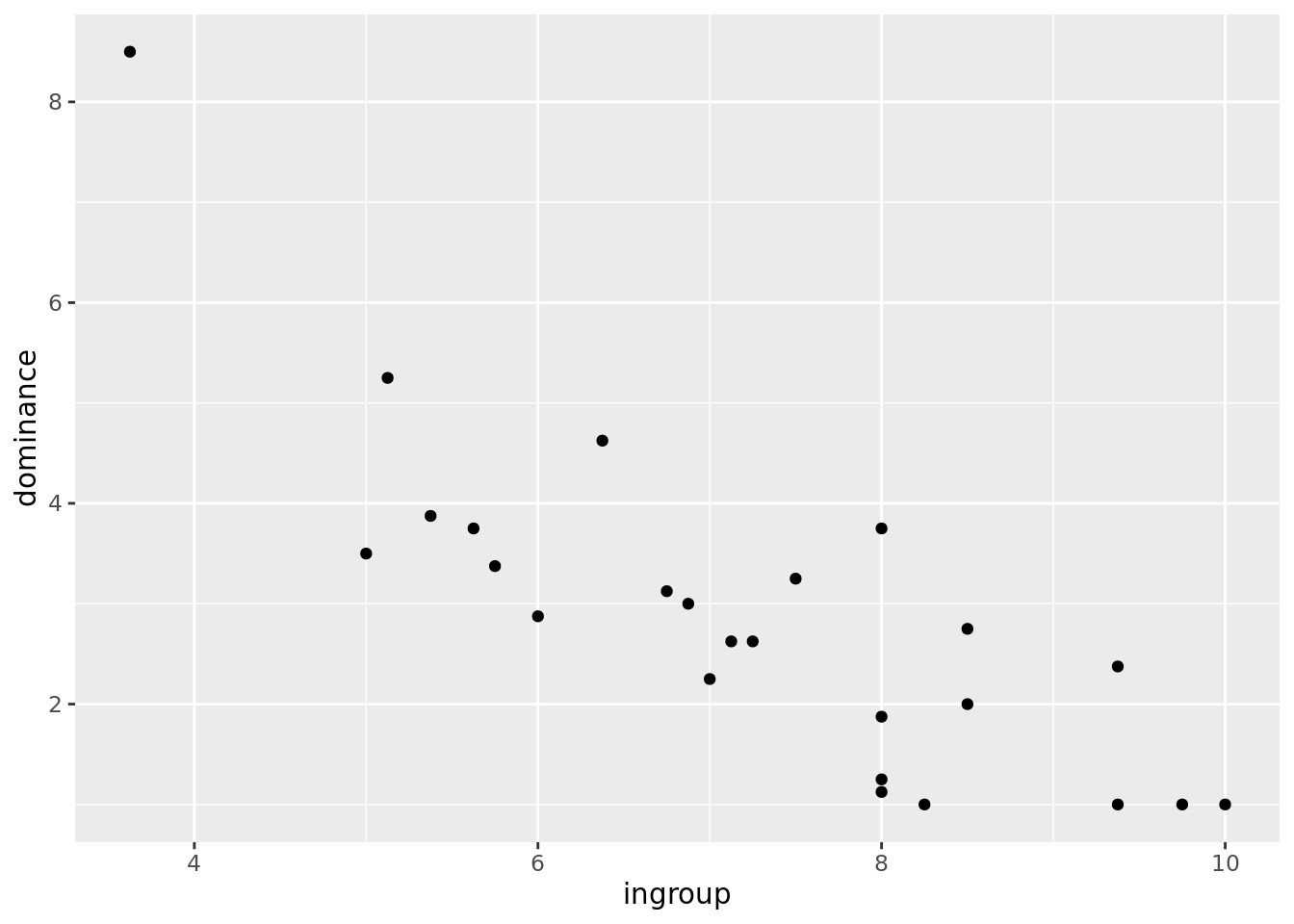-0.8196067
Bayes factor analysis
--------------
 Alt., r=0.333 : 10578.51 ±0%

Against denominator:
Null, rho = 0
---
Bayes factor type: BFcorrelation, Jeffreys-beta*

## The End!

If you’re able to complete the above exercise on your own, you’re all set! If not, ask for help in class, and/or work through the Absolute Beginners’ Guide to R